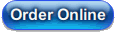# ARCH/GARCH and Volatility E-Course (2nd Edition)

This workbook is based upon the content of the RATS e-course on ARCH/GARCH and Volatility Models, offered in Fall 2012. Over the years, GARCH models have probably been the second most common application of the RATS software to appear in published articles (after Vector Autoregressions). Roughly half the course concerns the use of the existing GARCH instruction—determining the best specification, handling the estimation and doing tests of the adequacy of the model. The second half examines various extensions to the GARCH framework that require either more general likelihood maximization (Chapters 7 and 8), simulation methods (Chapters 9 and 10), or both, with detailed discussions of replications of papers which have been popular downloads by RATS users. The final chapter covers Stochastic Volatility Models, which are similar to GARCH but more complicated technically.

The second edition adds over 50 pages. Some changes reflect improvements to the GARCH instruction over the last few years, such as the new STDRESIDS and FACTORBY options for doing multivariate standardized residuals, and the new DENSITY and PARMSET options for using non-standard densities.

In addition, there is new or expanded coverage of

• ARMA models for the mean
• evaluating GARCH variance forecasts
• variance shift dummies derived from ICSS
• use/abuse of "rolling window" estimates
• generation of VECH representations for BEKK models
• tests for spillover in the mean and in the variance
• DCC models
• Variance Impulse Response Functions both closed form (for models that allow them) or through simulations (for models that don't)
• methods for handling extreme outliers
• GARCH-X models, particularly as they apply to BEKK
• VECM-GARCH models
• computing and displaying time-varying hedge ratios and portfolio weights
• Cermeño-Grier-style "panel GARCH" models

### Workbook Contents

(358 pages, 37 examples)

#### 1 Introduction

1.1 The Example
1.2 Tips and Tricks
Example 1.1 Simple Volatility Estimates

#### 2 Preliminary Analysis of Returns Data

2.1 Robust Sample Statistics
2.2 Extending to Multiple Series
2.3 Creating a Table
2.4 Tips and Tricks
Example 2.1 Summary Statistics Allowing for Serial Dependence
Example 2.2 Summary Statistics for Multiple Countries
Example 2.3 Summary Statistics with Table Generation

#### 3 Univariate ARCH and GARCH Models

3.1 Introduction
3.2 The Example
3.3 Choosing the Mean Model
3.4 Testing for ARCH Effects
3.5 Maximum Likelihood Estimation with Gaussian errors
3.6 Diagnostics for univariate ARCH models
3.7 Maximum Likelihood Estimation with Non-Gaussian Errors
3.8 QMLE Estimation
3.9 GARCH Models
Example 3.1 ARCH Model: Preliminaries
Example 3.2 ARCH Model: Estimation
Example 3.3 GARCH Model: Estimation

#### 4 More on Univariate GARCH Models

4.1 Forecasting
4.1.1 Evaluating Variance Forecasts
4.2 Stationarity
4.3 Stability Tests
4.4 Variance Equation Dummies
4.5 GARCH-M
4.6 Alternative Variance Models
4.7 Asymmetry
4.8 Rolling Samples
Example 4.1 Univariate Model Forecasting
Example 4.2 Stability Tests
Example 4.3 GARCH-M, EGARCH and Asymmetry

#### 5 Multivariate GARCH: Basics

5.1 Preliminaries
5.2 GARCH Instruction
5.3 Diagnostics
5.4 VECH, DVECH and BEKK Models
5.4.1 Diagonal VECH (Standard) Model
5.4.2 BEKK Model
5.5 Spillover
5.6 CC Models: Constant Correlation
5.7 DCC Models: Dynamic Conditional Correlation
5.8 RATS Tips and Tricks
5.8.1 Graphics with Multiple Series
5.8.2 Fancy Table of Diagnostics
Example 5.1 Multivariate GARCH: Preliminaries/Diagnostics
Example 5.2 Multivariate GARCH: DVECH Estimates
Example 5.3 Multivariate GARCH: BEKK Estimates
Example 5.4 Multivariate GARCH: Spillover Tests
Example 5.5 Multivariate GARCH: CC Estimates
Example 5.6 Multivariate GARCH: DCC Estimates

#### 6 More on Multivariate GARCH

6.1 Forecasting
6.2 Volatility Impulse Response Functions
6.2.1 Extensions of VIRF
6.3 Asymmetry
6.4 GARCH-X
6.5 Cointegrated Variables
6.6 Hedge Ratios and Related Calculations
Example 6.1 Multivariate GARCH: Forecasting
Example 6.2 Volatility Impulse Responses
Example 6.3 Multivariate GARCH with Asymmetry or Variance Dummies
Example 6.4 VECM-GARCH with X Regressor
Example 6.5 Hedge Ratios and Portfolio Weights

#### 7 Extensions Using MAXIMIZE-Univariate Models

7.1 A Simple Example
7.2 GMM Estimation
7.3 GARCH Model with Multiplicative Factor
Example 7.1 Maximum Likelihood Estimates of Restricted GARCH Model
Example 7.2 GMM Estimates of Heteroscedastic Model
Example 7.3 GARCH Model with Multiplicative Level Effect

#### 8 Extensions Using MAXIMIZE-Multivariate Models

8.1 Preparing the Data and Model
8.2 Panel GARCH
8.3 Non-standard Density
8.4 Structural VAR with GARCH
Example 8.1 Panel GARCH
Example 8.2 GARCH Model with Multivariate Skew-t Density
Example 8.3 Structural VAR-GARCH

#### 9 Simulations and Bootstrapping

9.1 Tools
9.2 Value-at-risk (VaR) Calculations-Univariate
9.3 Value-at-risk (VaR) Calculations-Multivariate
9.4 Variance Impulse Responses by Simulation
9.5 RATS Tips and Tricks
Example 9.1 VaR Calculations for a Univariate Model
Example 9.2 VaR Calculations for a Multivariate Model
Example 9.3 VIRF Calculations for with Asymmetry

#### 10 Simulation Methods for Model Inference

10.1 Bootstrapping
10.2 Monte Carlo Methods
10.2.1 Importance Sampling
10.2.2 Markov Chain Monte Carlo
10.2.3 MCMC Estimation of DCC Correlations
10.3 RATS Tips and Tricks
Example 10.1 Bootstrapping
Example 10.2 Univariate Markov Chain Monte Carlo Estimation
Example 10.3 Markov Chain Monte Carlo Estimation: Multivariate Model

#### 11 GARCH Models with Macroeconomic Data

11.1 An SVAR-GARCH-M model
11.2 Impulse Responses in an SVAR-GARCH-M Model
11.2.1 Error Bands
Example 11.1 SVAR-GARCH-M, Estimation
Example 11.2 SVAR-GARCH, Impulse Responses

#### 12 Stochastic volatility models

12.1 State-Space Approximation
12.2 Gibbs Sampling
Example 12.1 Stochastic Volatility: State-Space Approximation
Example 12.2 Stochastic Volatility: Gibbs Sampling

#### A Probability Distributions

A.1 Univariate Normal
A.2 Univariate Student t
A.3 Gamma Distribution
A.4 Inverse Gamma Distribution
A.5 (Scaled) Inverse Chi-Squared Distribution
A.6 Multivariate Normal
A.7 Multivariate Student (t)
A.8 GED distribution